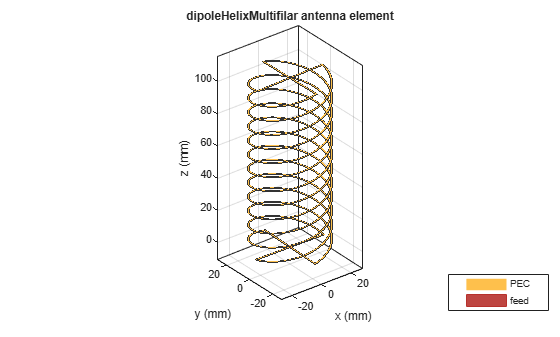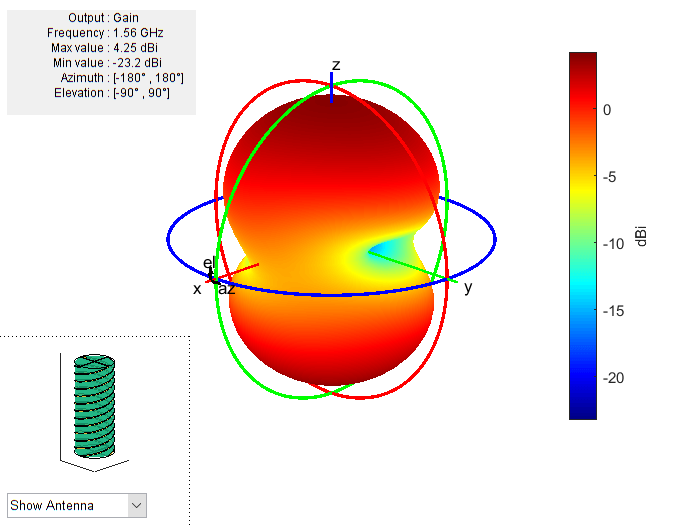# dipoleHelixMultifilar

Create balanced bifilar or quadrafilar dipole helix antenna without circular ground plane

## Description

The `dipoleHelixMultifilar` object creates a balanced bifilar or quadrafilar helix antenna without a circular ground plane. You can create both short-circuited and open-ended dipole helix multifilar antennas. Bifilar and quadrafilar helix antennas are used in aerospace and defense applications.

The width of the strip is related to the diameter of an equivalent cylinder by the equation

`$w=2d=4r$`

where:

• w is the width of the strip.

• d is the diameter of an equivalent cylinder.

• r is the radius of an equivalent cylinder.

For a given cylinder radius, use the `cylinder2strip` utility function to calculate the equivalent width. The default helix antenna is end-fed. The circular ground plane is on the X-Y plane. Helix antennas are used commonly in axial mode. In this mode, the helix circumference is comparable to the operating wavelength, and the helix has maximum directivity along its axis. In normal mode, the helix radius is small compared to the operating wavelength. In this mode, the helix radiates broadside, that is, in the plane perpendicular to its axis. The basic equations for the helix are

$\begin{array}{l}x=r\mathrm{cos}\left(\theta \right)\\ y=r\mathrm{sin}\left(\theta \right)\\ z=S\theta \end{array}$

where:

• r is the radius of the helical dipole.

• θ is the winding angle.

• S is the spacing between turns.

For a given pitch angle in degrees, use the `helixpitch2spacing` utility function to calculate the spacing between the turns in meters.## Creation

### Syntax

``ant = dipoleHelixMultifilar``
``ant = dipoleHelixMultifilar(Name,Value)``

### Description

example

````ant = dipoleHelixMultifilar` creates a bifilar or quadrafilar helix antenna without a circular ground plane. The default multifilar helical antenna is end-fed. The default helix operates around 2 GHz.```

example

````ant = dipoleHelixMultifilar(Name,Value)` sets properties using one or more name-value pairs. For example, `ant = dipoleHelixMultifilar('Radius',28e-03)` creates a multifilar helix with turns of radius `28e-03` m. Enclose each property name in quotes.```

## Properties

expand all

Number of helical elements, specified as a `4` or `2`. Two elements create a bifilar dipole helix antenna, and four elements create a quadrafilar dipole helix antenna.

Example: `'NumArms',2`

Example: `ant.NumArms = 2`

Data Types: `double`

Radius of the turns, specified as a positive real scalar meter.

Example: `'Radius',28e-03`

Example: `ant.Radius = 28e-03`

Data Types: `double`

Width of the strip, specified as a positive real scalar in meters.

Example: `'Width',0.2`

Example: `ant.Width = 0.2`

Data Types: `double`

Number of turns, specified as a scalar integer.

Example: `'Turns',4`

Example: `ant.Turns = 4`

Data Types: `double`

Spacing between the turns, specified as a positive real scalar in meters.

Example: `'Spacing',7.5e-2`

Example: `ant.Spacing = 7.5e-2`

Data Types: `double`

Status of ends of the helix, specified as `0` or `1`. By default, the `dipoleHelixMultifilar` is short circuited. Setting the property to `0` makes the helix antenna an open circuit.

Example: `'ShortEnds',0`

Example: `ant.ShortEnds = 0`

Data Types: `double`

Direction of helix turns (windings), specified as `CW` or `CCW`.

Example: `'WindingDirection','CW'`

Example: `ant.WindingDirection = 'CW'`

Data Types: `char` | `string`

Lumped elements added to the antenna feed, specified as a lumped element object handle. You can add a load anywhere on the surface of the antenna. By default, the load is at the origin. For more information, see `lumpedElement`.

Example: `'Load',lumpedelement`. `lumpedelement` is the object handle for the load created using `lumpedElement`.

Example: ```ant.Load = lumpedElement('Impedance',75)```

Data Types: `double`

Tilt angle of the antenna, specified as a scalar or vector with each element unit in degrees. For more information, see Rotate Antennas and Arrays.

Example: `'Tilt',90`

Example: `ant.Tilt = 90`

Example: `'Tilt',[90 90]`,`'TiltAxis',[0 1 0;0 1 1]` tilts the antenna at 90 degrees about the two axes defined by the vectors.

Note

The `wireStack` antenna object only accepts the dot method to change its properties.

Data Types: `double`

Tilt axis of the antenna, specified as:

• Three-element vector of Cartesian coordinates in meters. In this case, each coordinate in the vector starts at the origin and lies along the specified points on the X-, Y-, and Z-axes.

• Two points in space, each specified as three-element vectors of Cartesian coordinates. In this case, the antenna rotates around the line joining the two points in space.

• A string input describing simple rotations around one of the principal axes, 'X', 'Y', or 'Z'.

Example: `'TiltAxis',[0 1 0]`

Example: `'TiltAxis',[0 0 0;0 1 0]`

Example: `ant.TiltAxis = 'Z'`

Note

The `wireStack` antenna object only accepts the dot method to change its properties.

Data Types: `double`

## Object Functions

 `show` Display antenna or array structure; display shape as filled patch `axialRatio` Axial ratio of antenna `beamwidth` Beamwidth of antenna `charge` Charge distribution on metal or dielectric antenna or array surface `current` Current distribution on metal or dielectric antenna or array surface `design` Design prototype antenna or arrays for resonance at specified frequency `EHfields` Electric and magnetic fields of antennas; Embedded electric and magnetic fields of antenna element in arrays `impedance` Input impedance of antenna; scan impedance of array `mesh` Mesh properties of metal or dielectric antenna or array structure `meshconfig` Change mesh mode of antenna structure `optimize` Optimize antenna or array using SADEA optimizer `pattern` Radiation pattern and phase of antenna or array; Embedded pattern of antenna element in array `patternAzimuth` Azimuth pattern of antenna or array `patternElevation` Elevation pattern of antenna or array `returnLoss` Return loss of antenna; scan return loss of array `sparameters` S-parameter object `vswr` Voltage standing wave ratio of antenna

## Examples

collapse all

Create and view a default multifilar helical dipole antenna.

`ant = dipoleHelixMultifilar`
```ant = dipoleHelixMultifilar with properties: NumArms: 4 Radius: 0.0220 Width: 1.0000e-03 Turns: 3 Spacing: 0.0350 ShortEnds: 1 WindingDirection: 'CCW' Tilt: 0 TiltAxis: [1 0 0] Load: [1x1 lumpedElement] ```
`show(ant)`Create and view a quadrafilar helical dipole antenna with turn radius of 28 mm and strip width of 1.2 mm.

```ant = dipoleHelixMultifilar('Radius',28e-3,'Width',1.2e-3,'Turns',4); show(ant)```Plot the radiation pattern of the helical dipole at 1.8 GHz.

`pattern(ant,1.8e9);`